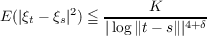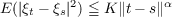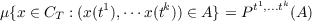#### Vol. 19, No. 1, 1966

 Download this articleFor screen For printingRecent Issues Vol. 325: 1  2 Vol. 324: 1  2 Vol. 323: 1  2 Vol. 322: 1  2 Vol. 321: 1  2 Vol. 320: 1  2 Vol. 319: 1  2 Vol. 318: 1  2Online Archive Volume: Issue:The Journal Subscriptions Editorial Board Officers Contacts Submission Guidelines Submission Form Policies for Authors ISSN: 1945-5844 (e-only) ISSN: 0030-8730 (print) Special Issues Author Index To Appear Other MSP Journals
Sample function regularity for Gaussian processes with the parameter in a Hilbert space

### Peggy Strait

Vol. 19 (1966), No. 1, 159–173
##### Abstract

In this note, Gaussian processes {ξt;t H} where H is the Hilbert space l2 are considered. It is shown that if T is a compact subset of a set of the form {(t1,t2,,tn,) : an tn an + 12n,(a1,a2,an,) H} (thus including all compact subsets of N-dimensional Eulidean space), and there exists constants δ > 0 and K > 0 such thatfor t,s in H, then almost all sample functions of the process are continuous on T. Furthermore, if there are constants α > 0 and K such thatfor all t,s in H, then “almost all” sample functions of the process are Lipschitz-β continuous on T for 0 < β < α∕2. The phrase “almost all” is used in the sense that the process defines a probability measure μ on the space CT of continuous or Lipschitz-β continuous functions on T, such that for any k points t1,t2,tk in T and any Borel set A in k-dimensional Euclidean space Rkwhere Pt1,tk is the probability measure defined by the random vector {ξt1,ξtk}. In the case where the process {ξt : t H} is separable and is separated by the set of dyadic numbers in H, then the phrase “almost all” as defined here takes on the usual meaning.

In application, it is shown that the Brownian process in a Hilbert space defined by Paul Levy satisfies the latter condition for α = 1. Thus almost all sample functions are Lipschitz-β continuous on T for 0 < β < 12 if T is a compact set of the form described above. Furthermore, it is shown that Levy’s result that almost all sample functions of this process are discontinuous in the Hilbert sphere may be extended to arbitrary noncompact subsets of the form T = {(t1,t2,,tn,) : an tn bn}.

Primary: 60.40
##### Milestones